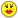+0

# help

-1
204
5

Can you help?Apr 19, 2019

#1
+2

wow, I totally messed this one up... have to stop answering these when I'm drinking!Let's start again shall we

$$P[k] = \dfrac{\dbinom{20}{k}\dbinom{10}{3-k}}{\dbinom{30}{3}}\\ P[(0,1,2,3)] = \large \left(\frac{6}{203},\frac{45}{203},\frac{95}{203},\frac{57}{203}\right)$$

$$E[X] = 0\cdot \dfrac{6}{203} + 1 \cdot \dfrac{45}{203}+2\cdot \dfrac{95}{203}+3\cdot \dfrac{57}{203} =2$$

.
Apr 19, 2019
edited by Rom  Apr 22, 2019
edited by Rom  Apr 22, 2019
edited by Rom  Apr 22, 2019
#2
+2

One question,Rom...[I'm not too familiar with this....]

Don't we have

(0)(57/203) + 1(95/203) + 2(45/203) + 3(6/203)   by  the summation formula???Apr 19, 2019
#3
+1

oops we sure do

Rom  Apr 22, 2019
#4
+2

Rom, the RHS your equation was correct; it’s the LHS (formula) that has the error.

$$E[X] = \underbrace {\sum \limits_{k=0}^3 k \cdot P[k]}_{LHS}= \frac{57}{203} + 2 \cdot \frac{95}{203} + 3 \cdot \frac{45}{203} + 4 \cdot \frac{6}{203} = 2\\$$

The general formula for expectation:

$${E} [X]=\sum \limits _{i=1}^{k}x_{i} \cdot p_{i} ,|, \small \text{Where (k) is the total number of events, and (i) is its index, multiplied by its probability.}$$

You (intuitively) corrected for this on the RHS. I assume the different use of the (k) variable derailed your intent. In the moment, we intellectually know that while every kissbegins with (k), not every kissis the same. But... it’s easier to analyze that after the fact, though.----------

For this question, it’s easy to verify that two (2) is the expected number of juniors in the committee: The 20 juniors represent (2/3) of the total sample space of 30.  (2/3) of (a random sample of) three (3) = 2; the expected number of juniors.

GA

Apr 22, 2019
edited by GingerAle  Jun 30, 2019
#5
+1

see edited first post.  I had reversed the number of juniors and seniors in deriving the distribution.

Rom  Apr 22, 2019